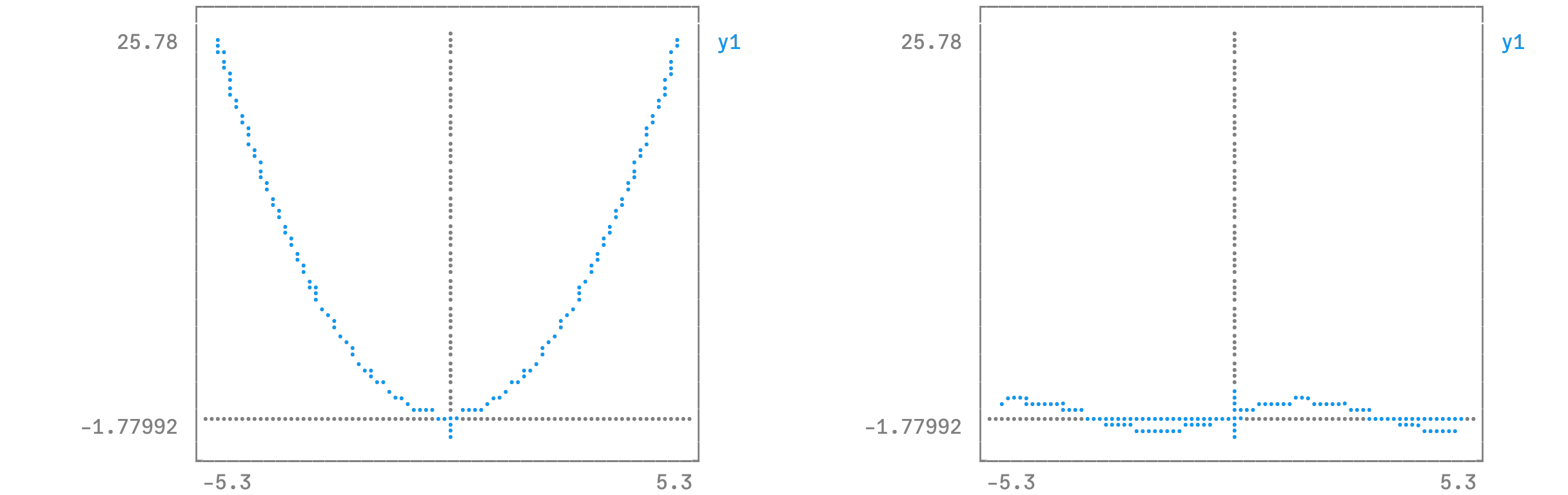using Plots
unicodeplots()
x = -5:0.1:5
plot(plot(x, (x->begin
x ^ 2
end)), plot(x, (x->begin
sin(x)
end)), layout = 2, link = :y)